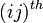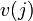# Adjacency matrix

Jump to: navigation, search

## Definition

### For a finite undirected graph

Supposeis a finite undirected graph. Letbe the size of the vertex set. Fix a bijective correspondence. The adjacency matrix ofis defined as thematrix whoseentry is:

• 0 if there is no edge betweenand• 1 if there is an edge betweenandNote that this matrix is well defined only after we fix the bijection. Changing the bijection has the effect of conjugating the adjacency matrix by a permutation matrix obtained by composing one bijection with the inverse of the other. In other words, we re-label the rows and re-label the columns in the same manner. In particular, the characteristic polynomial as well as the multiset of eigenvalues do not depend on the choice of ordering of vertices.

### For a finite undirected graph allowing parallel edges, loops, and weights

• If we allow loops (but no parallel edges or weights), the definition does not change, but it may now be possible to have 1s on the diagonals at the entries corresponding to loops.
• If we allow parallel edges, then theentry is taken to be the number of edges betweenand.
• If we allow weights, we could use the weighted adjacency matrix instead.

### For a finite directed graph

Supposeis a finite undirected graph. Letbe the size of the vertex set. Fix a bijective correspondence. The adjacency matrix ofis defined as thematrix whoseentry is:

• 0 if there is no edge fromto• 1 if there is an edge fromto### For a finite directed graph allowing parallel edges, loops, and weights

• If we allow loops (but no parallel edges or weights), the definition does not change, but it may now be possible to have 1s on the diagonals at the entries corresponding to loops.
• If we allow parallel edges, then theentry is taken to be the number of edges fromand.
• If we allow weights, we could use the weighted adjacency matrix instead.

## Facts

• The adjacency matrix of a finite undirected graph is a symmetric matrix. This remains true even if we allow loops and parallel edges.
• The adjacency matrix of a finite undirected graph has entries only 0s and 1s. This remains true even if we allow loops but is no longer true if we allow parallel edges.
• The adjacency matrix of a finite undirected graph has 0s throughout the diagonal. This remains true even if we allow parallel edges but is no longer true if we allow loops.